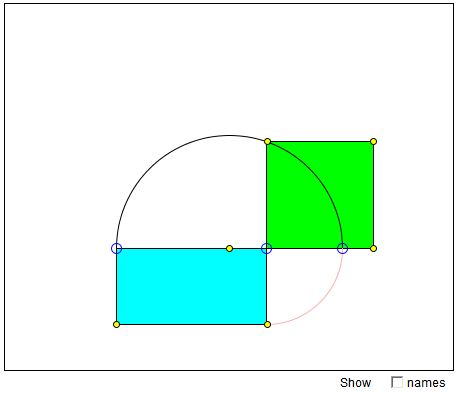### If you are reading this, your browser is not set to run Java applets. Try IE11 or Safari and declare the site https://www.cut-the-knot.org as trusted in the Java setup.What if applet does not run?

Explanation### Squaring a Rectangle

The applet suggests a construction [Heath] of a square equal in area to a given rectangle (Euclid II.14). The two shapes are equidecomposable, as are any two polygons of equal area (see Wallace-Bolyai-Gerwien Theorem.) Thus we already know how to solve the problem of squaring a rectangle. The applet shows a different, yet no less constructible, way of achieving the same goal. The construction is based on an appearance of the geometric mean in the right triangle. The applet follows Euclid VI.13 which naturally differs from the construction in Euclid II.14.Let ABDE be a rectangle. Construct C on the extension of AB such that BC = BD. Form a semicircle on AC as a diameter. Let F be the point of intersection of BD and the semicircle. Then

 BF2 = AB·BC = AB·BD,

which is what is required.

### References

1. T. L. Heath, Euclid: The Thirteen Books of The Elements, Dover, 1956